## Calculating Working Days in Excel

In this article, we will examine how to work with and calculate working days in Excel. This includes calculating the number of working days between two dates and also the other way around, we will also figure out what end date will be x number of days after a particular start date.

This can come very useful when it comes to project management where we often need to be mindful of how many days we have left before deadline. Or we may need to work out what the expected project end date should be after working out how many working days we need.

## No. of Working Days Between Two Dates

In Excel, there is a built-in function which calculates the number of working days between two dates. According to Excel, working days are Mondays to Fridays. This is the NETWORKDAYS function. There are only two mandatory fields:

• Start Date
• End Date

Let’s go through a simple example below: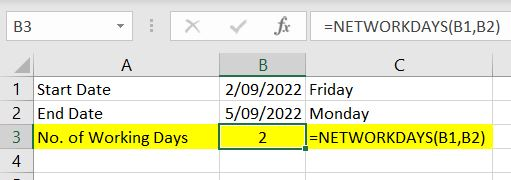=NETWORKDAYS(B1,B2)

The function returns 2 as the result. The two working days are 02/09/2022 and 05/09/2022. Excel knows to not count 03/09/2022 and 04/09/2022 because they are Saturday and Sunday. And as we can see in the example above, the start date and end date are inclusive. This can be a useful formula in project management and in workforce planning.

It is not all there is to the NETWORKDAYS function. When we need to calculate the number of working days over a long period of time, it will be even more useful if we can take holidays into account. Luckily in the NETWORKDAYS function, there is an optional field:

• Holidays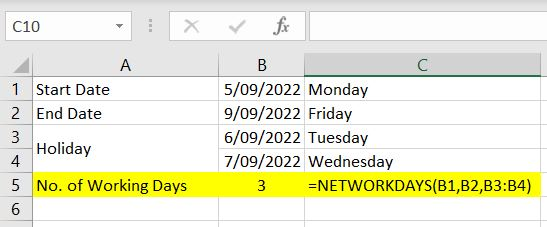=NETWORKDAYS(B1,B2,B3:B4)

In this example, we want to calculate the number of working days between 5th September 2022 to 9th September 2022. That’s Monday to Friday so there are 5 working days. However we’ve specified that 06th and 07th are holidays. Hence Excel removed those two dates as working days and returned 3 as the result.

Using this function, we can calculate total number of working days in a year by having 1st January as the start date, 31st December as the end date and also list out all the holidays for the year and reference them in the formula.

### End Date Prior to Start Date

With NETWORKDAYS function, it is possible to have an end date that is before the start date. When that happens, Excel will return a negative number as a result: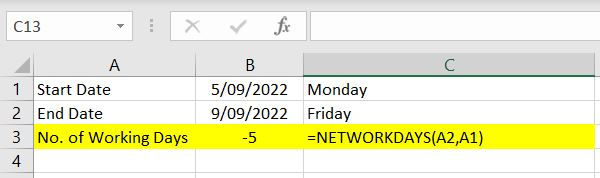## Date After Nth Working Days

Sometimes it works the other way around! Sometimes we have a start date and we know how many working days we have, then we need to work out what the end date should be. In Excel, there is a built-in function just for that – WORKDAY function. Again there are two mandatory fields:

• Start Date
• No. of Days

Again let’s start off with a simple example: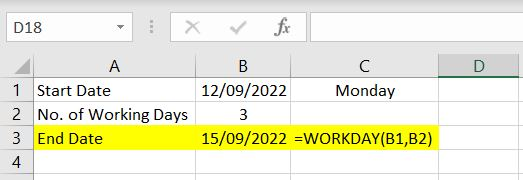=WORKDAY(B1,B2)

In this example, we have 12th September 2022 as the start date and we want to know the date 3 working days after this start date. Again this function will know to skip weekends: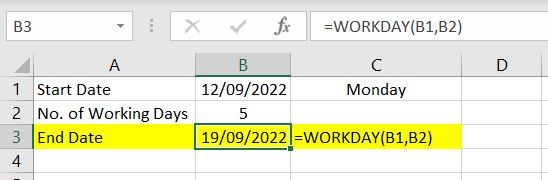If we have Monday as the start date, 5 working days later, it will be the following Monday (19th September).

Once again, we can take holidays into account with this function. It is very similar to the NETWORKDAYS function. There’s an optional field in the WORKDAY function which allows for holidays to be taken into account.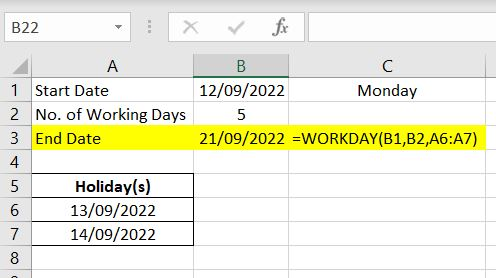=WORKDAY(B1, B2, A6:A7)

In the example above, we’ve put 13th and 14th of September as holidays and referenced them in WORKDAY function. Hence the end date has been pushed from 19th to 21st September.

Another similarity between the NETWORKDAYS and the WORKDAY functions is that they can both calculate negative no. of working days: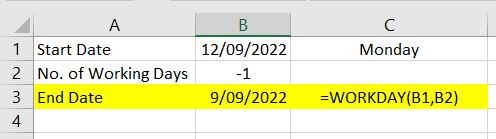=WORKDAY(B1, B2)

With 12th September as the start date (Monday), if we want to find the working day before that, it’ll be the previous Friday – 9th September.

As we can see, WORKDAY and NETWORKDAYS functions are simple to use but can be very useful. It can save a lot of time in a work environment. If you can think of other ways to use these functions or if there’s anything we’ve missed, please leave a comment below!